We detect you are using an unsupported browser. For the best experience, please visit the site using Chrome, Firefox, Safari, or Edge. X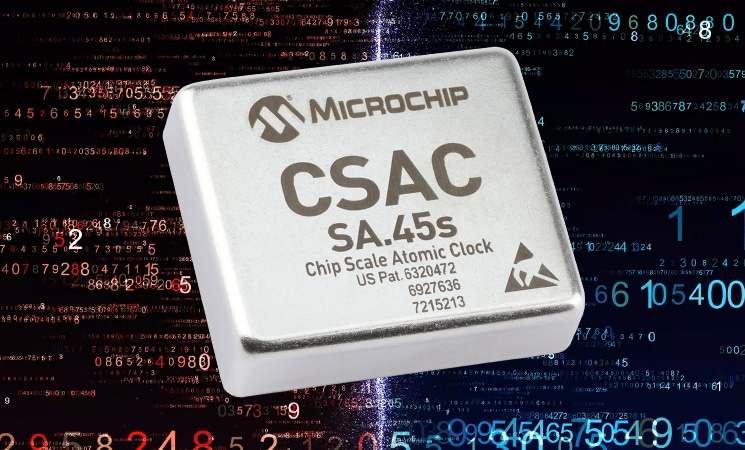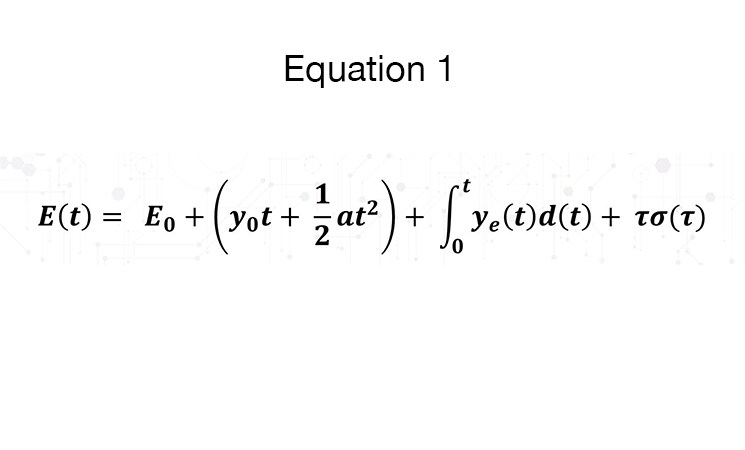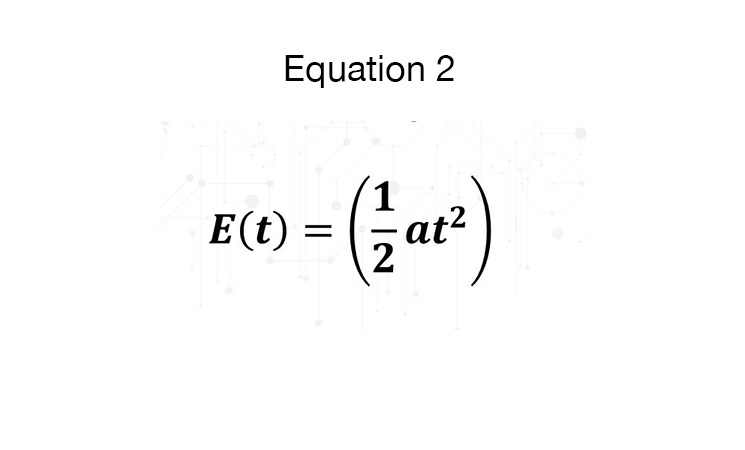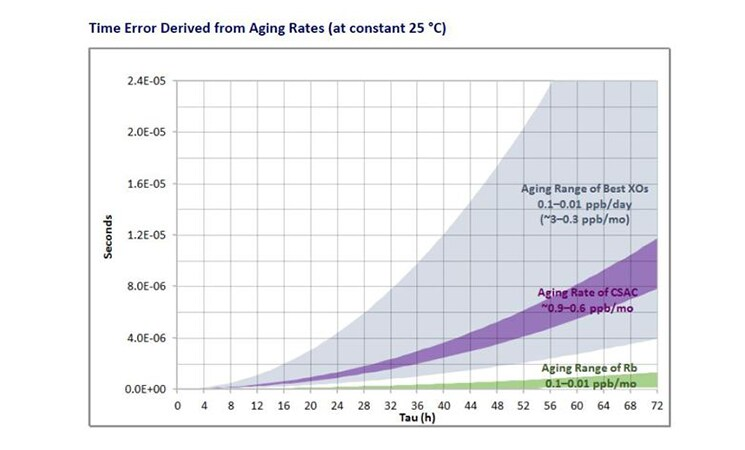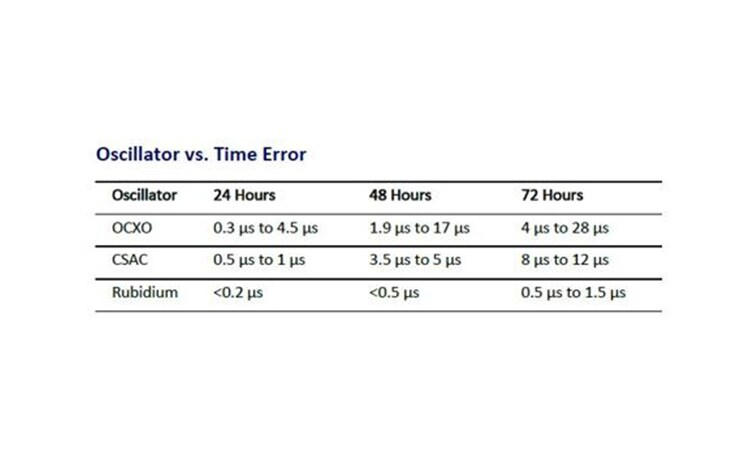# Chip Scale Atomic Clocks: Effects on Timing Error – Elapsed Time (Part 2 of 4)

In this article on the Chip Scale Atomic Clock (CSAC) performance during rapid temperature change, learn how time error can be calculated from published aging rates.

In this article, I explain how time error can be calculated from published aging rates. Given the small size and power consumption of the CSAC, the calculated holdover performance is impressive.

Time error can be calculated from published aging rates, as shown in References. Taking the result of their derivation, the timing error accumulation over time is given as follows in Equation 1

where,

E(t): Time error accumulation at time t after initial synchronization

a: Clock frequency drift (or aging) rate

E0: Initial time error at t = 0

Ei(t): Fractional frequency offset due to environmental effects (such as temperature)

y0: Initial fractional frequency at t = 0

ε(t): Error due to random fractional frequency fluctuations

For simplification purposes, we have assumed zero initial phase or frequency offset and zero

environmental perturbation, which reduces the equation to what can be viewed in Equation 2.

The following graph and table show predicted time error over 72 hours of the CSAC, high-performance OCXOs, and Rubidium atomic oscillators. CSAC will accumulate ~1 μs in 24 hours, ~5 μs in 48 hours, and 8 μs to 12 μs in 72 hours. These calculations were made based on typical observed aging rates of 0.6 ppb /mo to 0.9 ppb/mo.

Note: Throughout this series of articles, RF output (10 MHz) performance of the CSAC will be shown. The CSAC 1PPS output is directly derived from the RF output, so it should be expected that the 1PPS would behave in a similar manner.

View the Time Error Derived from Again Rates (at constant 25 °C).

View the Oscillator vs. Time Error chart.

In the next article of this series, I’ll write about the effects on timing error: Temperature.

Read part 1 of 4 on the Chip Scale Atomic Clock performance during rapid temperature change.

To read the complete white paper, go here now.

Will Krzewick, Oct 20, 2020